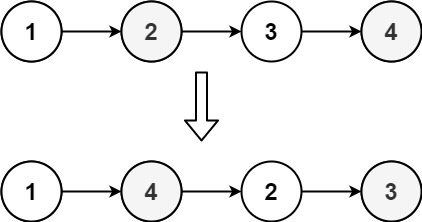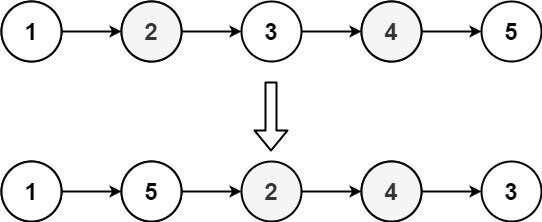### LeetCode• ㊗️
• 大家
• offer
• 多多！

## Problem

You are given the head of a singly linked-list. The list can be represented as:

$L_0 → L_1 → … → L_{n - 1} → L_n$

Reorder the list to be on the following form:

$L_0 → L_n → L_1 → L_{n - 1} → L_2 → L_{n - 2} → …$

You may not modify the values in the list’s nodes. Only nodes themselves may be changed.

Example 1:Input: head = [1,2,3,4]
Output: [1,4,2,3]


Example 2:Input: head = [1,2,3,4,5]
Output: [1,5,2,4,3]


Constraints:

• The number of nodes in the list is in the range $[1, 5 * 10^4]$.
• $1 <= Node.val <= 1000$

## Code

/**
* public class ListNode {
*     int val;
*     ListNode next;
*     ListNode() {}
*     ListNode(int val) { this.val = val; }
*     ListNode(int val, ListNode next) { this.val = val; this.next = next; }
* }
*/
class Solution {

ListNode temp = null;

while(fast != null && fast.next != null){
temp = slow;
slow = slow.next;
fast = fast.next.next;
}

temp.next = null;

ListNode l2 = reverse(slow);
}

ListNode prev = null;
}

return prev;
}

private void merge(ListNode l1, ListNode l2){
while(true){
ListNode n1 = l1.next;
ListNode n2 = l2.next;
l1.next = l2;
if(n1 == null) return;
l2.next = n1;
l1 = n1;
l2 = n2;
}
}
}